Détails du magazine
Format
Magazine
eISSN
1891-5469
Première parution
01 Jan 2010
Périodicité
1 fois par an
Langues
Anglais
Accès libre

# Impedance of tissue-mimicking phantom material under compression

###### Reçu: 27 Nov 2012
Détails du magazine
Format
Magazine
eISSN
1891-5469
Première parution
01 Jan 2010
Périodicité
1 fois par an
Langues
Anglais

Note on Terminology: Throughout this article some variables are used that have different meanings in different disciplines. Examples include ρ (meaning density here, not resistivity), σ (meaning stress here, not conductivity), E (meaning the elastic modulus here, not electric field strength), and ε (meaning strain here, not permittivity). Special care should be taken to understand the variables in context. Definitions of every variable are given throughout the article.

Introduction

Bioimpedance is the term used to describe the study of tissues subject to external electrical excitation. It is distinct within the field of electrophysiology in that it is primarily concerned with ascertaining the electrical properties of biological tissues (whether living, dying, or dead) by inducing a current, measuring the response, and inferring the underlying physiological mechanisms. The response typically measured is the difference in electrical potential, voltage, between two points. Applying the complex version of Ohm’s law, the indirect measure of impedance can be found as the ratio of voltage to current. By properly measuring passive electrical characteristics, it becomes possible to understand biological systems in a unique and useful way.

With this knowledge engineers and scientists have found much to discover and draw upon. From the analysis of single cells (1) and cultures (2) to tissues (3) and organs (4) to whole bodies (5) and across populations (6), bioelectrical impedance has proven its value in quantifying physiology time and again. While the spatial scale of the samples ranges from cell membranes to human populations, the temporal range is not nearly as robust. Typical bioimpedance measurements are often around several seconds. Hidden behind the veil of these seconds are many important and interesting physiological events, not the least of which are changes in tissues under physical deformation. One of the most significant ways tissues become mechanically deformed is through compression. The impedance of a material under this type of deformation is the focus of our study.

Biological tissues can become subject to compression in several ways, including physiologically (pressure exerted on vessel walls during the cardiac cycle (7) and cellular mechanotransduction (8,9)), pathologically (traumatic injuries (10) clinically (edema monitoring for dialysis patients (11), electrosurgery (12), body water and fat detection (5), and soft-tissue healing techniques (13), and in basic research (optical clearing in tissues (14) and probe characterization (15). Even fields that would at first seem to fall far abreast of this specific discussion have added relevance given their synthesis of passive electrical properties with the mechanical properties of materials under examination including certain realms of agricultural research focused on meat quality assessment (16,17) and mineralogical investigations of rocks (18). All of this research suggests there is likely an underlying correlation between mechanical compression of a material and detectable changes in its electrical impedance. This agrees with our intuition because as we have said, impedance is meant to provide an indirect measurement of underlying material properties.

Despite the ever-present reality of compressed tissue (consider for a moment that at least one portion of your body is being compressed), there currently exists a dearth of literature studying the electrical impedance properties of biological samples under compression. As such a field investigating the correlative effects of tissue deformation and electrical impedance is much needed.

Of interest to such a field of study are the results of Gonzalez-Correa et al. which found that the resistivity of various tissues increased as the pressure of the measurement probe was increased (19, concluding that fluid loss from the extracellular compartment of the compressed tissue was response for this increased impedance. Confounding this result slightly is the fact that no evidence is provided in these experiments to show that the authors have accounted for the effects of proximity of the electrodes to the bottoms of their measuring devices (a known source of impedance dependence (20). Similarly experiments on bladder tissue have shown that probe pressure has a significant effect on measured impedance (21). In fact, the authors go on to reason that there is an optimal range of pressures to apply to particular tissues, with too little pressure not providing sufficiently reliable contact and too much pressure beginning to modify the tissue’s properties (22).

Working with near isotropic pig spleen tissue, Dodde et al. were the first to explicitly measure the electrical impedance of biological tissue under compression, finding that increased levels of compression correlated well with increases in intra- and extra-cellular resistances, when fit with the Cole-Cole model (23). While the aforementioned researchers believe that the loss of fluid is primarily responsible for the increase in impedance readings, all did so under steady-state conditions, disregarding the dynamic relaxation behavior of the tissue under consideration. In this study, we consider the relationship between the viscoelastic behavior of a phantom material – namely tofu – to its measured bioimpedance in real-time.

Tofu has become a popular phantom material in many fields of biological interest including elastography (24) and ultrasound imaging (25). Tofu, also referred to as bean curd, is a food made by pressing curds from coagulated soymilk into blocks of relative homogeneity. Coagulation of the soymilk is usually achieved in one of two ways, either through the use of salts (such as calcium sulfate or magnesium chloride) or through acids (glucono delta-lactone which is often used in the manufacturing of cheese being the most common). After coagulation, the curds are pressed together to form a porous matrix structure (26). Because many manufacturers have automated this process and have even begun to use electrical impedance spectroscopy to do so (27,28), tofu is becoming more and more popular as a widely available, consistent, and economically practical tissue-mimicking material for soft biological tissues 29.

In this paper we explore the mechanism by which the passive electrical properties of a quasi-biological phantom of soft tissues are altered by compression. We postulate that exodus of the conductive fluid from the sample results in a decrease in the sample’s overall admittance. Furthermore, we contend that this decrease in admittance is intrinsically related to the stress relaxation function of the sample as it is under compression. In testing the validity of this claim, we model tofu as a Maxwellian viscoelastic material (7, 30) and fit both stress and admittance relaxation to this model with a high degree of correlation. From these relationships we are able to describe the tofu’s passive electrical properties wholly from its mechanical properties. Within the given range of compressions we are able to describe the tofu sufficiently, though more advanced modeling is required. Finally, we show that tofu is a viable phantom for soft tissue compression studies utilizing bioimpedance techniques.

Modeling tofu as a bioimpedance phantom material
Fluid exodus

Typically bioimpedance models are described by a variation of a parallel circuit whose components represent the impedance of the suspending fluid in one branch and the suspended elements on the other branch opposite (Fig. 1). The total impedance of tofu, Z, is the parallel combination of two impedances: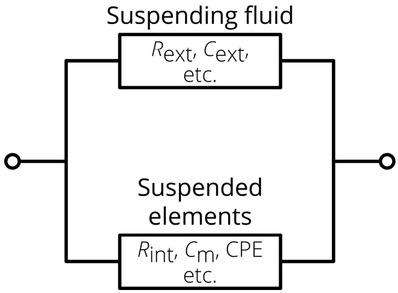The basic schematic diagram of many bioimpedance models consisting of parallel branches representing the suspended elements and the suspension in which they rest. In this case Rext and Cext would be the resistance and capacitance of the suspending fluid, respectively and Rint, Cm, and CPE would be the internal resistance of the suspended elements, the membrane capacitance and a constant phase element of the suspended elements, respectively. However, any components could be used along these branches.$Z=[1Z1+1Z2]−1$$$Z={{\left[ \frac{1}{{{Z}_{1}}}+\frac{1}{{{Z}_{2}}} \right]}^{-1}}$$

where, Z1 represents the impedance of the saline solution held within the porous structure of the bean curd, whose impedance is represented by Z2.

Tofu consists of these two independent electrically conducting components, with the solid porous structure made of compressed coagulated soymilk curds suspending the preserving fluid, usually saline. Saline solution has long been known to be a purely conductive (resistive) element (31) and its impedance, is described as a constant resistance, R1. For the porous bean curd structure, Li and Toyoda (27,28,32) have measured the impedance of soymilk as it coagulates, the procedure that leads to the creation of tofu. The authors used both a capacitor and a resistor in series to model the impedance measurements of coagulating milk, but only introduced the reactive element to account for the polarization of measurement probe itself. After a threshold frequency is passed (approximately 1 kHz), the coagulated soymilk’s impedance remains constant with increasing frequency, representing the bulk resistance. The branch representing the porous bean curd structure can therefore be modeled as a constant resistance, R2. The relative contribution of the two impedance elements from Eq. (1) is hypothesized to correspond to the amount of a component within the total sample at any given time. A simple way of phrasing this idea is to consider this relative contribution equal to ratio of the density of one component, ρi, – defined here as the mass of the component, mi, divided by the total volume, Vtot, of the sample (i.e., ρi = mi/Vtot) – to the overall density of the whole sample (ρtot = mtot/Vtot) as

$Z=1ρ1ρtotR1+1ρ2ρtotR2.−1$$$Z={{\left[ \frac{1}{\frac{{{\rho }_{1}}}{{{\rho }_{\text{tot}}}}{{R}_{1}}}+\frac{1}{\frac{{{\rho }_{2}}}{{{\rho }_{\text{tot}}}}{{R}_{2}}} \right]}_.^{-1}}$$

If R2 > R1, Eq. (2) predicts that the overall impedance of the system should increase as the contribution of (ρ2/ρtot)R2 increases or as the contribution of (ρ1/ρtot)R1 decreases, though for our model one will necessarily lead to the other. This trend agrees with the hypothesis that as the sample is compressed the more conductive fluid will be squeezed out of solid porous matrix of the bean curd thereby increasing the measured impedance. Viewed conversely, the admittance (the reciprocal of impedance) should decrease with time, as stress does, as the saline solution is removed. In this way, it should be possible to correlate the stress the sample is experiencing to its passive electrical properties.

Using tofu as a phantom allows us to approximate the mechanical behavior of soft tissues and track fluid loss without complicating the matter with the complex representation of impedance. This limits the applicability of this phantom to physiological situations where extracellular fluid and tissue density are the predominate factors of impedance changes. Such examples include typical tissue compressions (such as those experienced during sitting or standing) and lymphedema treatments. However, due to the purely resistive nature of tofu, it is incapable of accounting for all tissue situations, which would require some capacitive element. To this extent, tofu may be considered a tissue-mimicking phantom insofar as it is relegated to a specific subset of tissues undergoing a subset of possible compression mechanisms.

Mechanical properties

A necessary assumption to our mechanical model is that the relative contribution of the two components of the tofu samples is a function of stress relaxation, G(t), for a given strain level:

$ρ1ρ2=fGt.$$$\frac{{{\rho }_{1}}}{{{\rho }_{2}}}=f\left( G\left( t \right) \right)_.$$

If this were not the case – if there was no causal connection between the density ratios of the two components comprising the material under investigation and the stress relaxation of that material – then there would be no way of relating the measured passive electrical properties to the physical deformation of a sample. On the one hand, it is a very likely assumption (that impedance measurements reflect and underlying physical nature) as it is thoroughly baked into all bioimpedance research and has been successful thus far. On the other hand, the function in this particular case involves the flow of fluid through a deforming, quasi-biological, porous structure and is beyond the scope of this presentation. This study demonstrates that such a function does exist without necessarily describing its precise formulation.

The stress relaxation function chosen here is the Maxwell model represented as a simple spring and dashpot in series (Fig. 2). With constant strain and negligible creep observed, the model is suited for this initial investigation.

Assuming an instantaneous strain, ε0, is applied at a time, t = 0, the stress-strain relationship for the Maxwell model of viscoelasticity can be described as

$σ(t)=Eε0e−Eμt$$$\sigma \left( t \right)=E{{\varepsilon }_{0}}{{e}^{-\frac{E}{\mu }t}}$$

where σ(t) is the stress at a given time, E is the elastic modulus of the sample, and μ is the viscosity (relating force to displacement rate) (30). Furthermore, by dividing both sides by the initial strain, the stress relaxation function, G(t), for the Maxwell model becomes

$G(t)=Ee−Eμt.$$$G\left( t \right)=E{{e}^{-\frac{E}{\mu }t}}.$$

The relaxation time constant, τR, is defined as the ratio of the viscosity and the elastic modulus as

$τR=μE$$$\tau _R=\frac{\mu }{E}$$

leading to a concise expression of the model:

$Gt=Ee−tτR.$$$G\left( t \right)=E{{e}^{-\frac{t}{\tau_R}}}.$$

The relaxation function may be normalized to the peak stress (σmax, stress at t = 0) as

$Gnormt=σσmax1ε0=Eσe−t/τR,σ$$${{G}_{norm}}\left( t \right)=\frac{\sigma }{{{\sigma }_{\max }}}\frac{1}{{{\varepsilon }_{0}}}={{E}_{\sigma }}{{e}^{{-t}/{{{\tau _R,\sigma }}}\;}}$$

where σ is the stress, ε0 is the initial strain represented as the percentage of total compression (the ratio of the change in height to the initial height) placed on the tofu sample at t = 0, Eσ is the elastic modulus of the tofu as a function of peak stress, and τR is the characteristic stress relaxation time constant (an indicator of how long it takes for the system to settle).

More advanced models of viscoelasticity exist. Their relevance is discussed in the Conclusions section of this work. For the purposes of this investigation, however, the model will suffice and the parameters (the elastic modulus and the characteristic relaxation time constant) are satisfactory to describe any trends that arise.

Electrical properties

It is our contention that there will be a corresponding admittance relaxation curve to the stress relaxation equation presented above (Eq. (8)). This will serve to prove the contentions of many previous researchers (15,19,21-23) under more rigorous examination. Our purpose here is to show that tofu is a practical phantom of soft tissues for electrical impedance measurements. Lacking a capacitive element, its impedance is constant over a wide frequency range, enabling probe calibration and verification of experimental procedures investigating the compression of biological materials.

Materials and methods

The basic procedure outlined in this section was used to correlate tofu compression to impedance/admittance data. It was done to show the electrical impedance can be used to describe mechanical phenomena of biological materials. Two different experimental procedures were conducted: single frequency and multifrequency current injections. Because tofu exhibits constant impedance over the range of frequencies we tested (1 kHz to 300 kHz), only the single sine current injection method and results are reported here. As the single sine method had the lowest crest factor – the ratio of the peak amplitude of a signal to its root mean squared valued –of the two techniques, indicating a higher quality of alternating current, this technique is preferred. It is worth noting that a multifrequency technique is necessary for future explorations of biological samples with capacitive elements under compression.

A custom designed tetrapolar probe made of four 0.25 mm diameter platinum electrodes equally spaced 3.0 mm apart in a straight line encased in a 19 mm diameter acrylic cylinder (Fig. 3) was used to acquire impedance (23,33). Probe geometries have been shown to be optimally sensitive to impedance measurements (34,35). The impedance probe was mounted inline with a load cell (Omega LC8125) and both were attached to a linear stage (Siskiyou 200cri) used to compress the material under testing (tofu). A cylindrical acrylic chamber (25.4 mm inner diameter) was machined with a series of radial holes (3.8 mm in diameter) allowing the free flow of fluid and used to hold the tofu in place. At the bottom of the holding chamber was a pressure sensor (GE NPC-1210).

The impedance probe was fed through a custom circuit designed specifically for soft biological tissue impedance measurements (23). Characterization of the current-to-voltage converter circuit was done by passing a voltage signal through a known load in series with the input to obtain a known current. The input signal was compared to the output and the corresponding gain and phase shift attributes that resulted could then be factored out. The voltage-controlled current source was characterized by passing the whole frequency range of output current (100 Hz to 1 MHz) through a range of known resistances, including the fluid used to preserve the tofu. The measured output behavior of current was found as a function of frequency for each resistance load. Finally, the instrumentation amplifier circuitry was described by inputting a known differential voltage signal and comparing the output signal for gain and phase shift. The calibration necessary to offset each of the above elements was done at either the data acquisition phase or through post-processing. The circuit design as well as its printed circuit board realization can be found by consulting Dodde (23,33).

In factoring out impedance errors, the effects of stray capacitance on our bioimpedance measurements – a known source of error (36,37,38) – were removed using the method described by Buendia et al. (39,40). Also as a precaution, the effects of probe depth (20) were accounted for in post-processing analysis, though the measurements were not significantly influenced at the levels the experiments were conducted – impedance changes of <1% were observed so long as the probe was >7 mm from the bottom of the testing chamber.

The load and pressure sensors were connected to a noise-rejecting, shielded BNC connector block (BNC-2110) that was subsequently fed to a data acquisition card (NI PXI-6229). Motion of the linear stage was controlled through an NI PXI-7340 motion controller that gave. Each of the modules were housed within a NI PXI-1033 chassis and monitored and controlled via a custom LabVIEW program.

Cylindrical tofu specimens were obtained by cutting a block of (Naturally PreferredTM) extra firm, organic tofu approximately in half and punching out 22 mm diameter samples using a sharpened leather punch, which tended to give generally uniform tofu specimens (typically about 20±2 mm in height). The cylindrical samples were then kept in a container filled with the original preservation fluid. With samples at the ready, the program was begun and the bottom of the testing chamber was found by lowering the linear stage slowly (~10 μm/s) until the bottom was detected as the load sensor inline with the probe registering some amount of force (Fig. 3). The linear stage then lifted the probe out of the chamber to such a height that a sample could be placed easily. A sample was then chosen at random, its mass was found before compression, and it was placed into the sample holding chamber. Once there, the linear stage then lowered the probe onto the tofu sample until a detectable amount of current passed through, ensuring that as the tofu is compressed sufficient electrical contact would be maintained. As the top of the tissue is found through impedance detection, the height of the sample was recorded and the density of the sample before compression was found. If no problems were apparent at this point, the sample was injected with 60 μA RMS of current at 10 kHz and compressed to one of four initial strain levels, ε0, (10%, 20%, 30%, or 40% compression of total height) at random. Strains above 50% were not permissible, as the tofu would begin to break apart.

The pressure, load, and impedance were measured in real time (<0.1 s). Individual runs were measured over the course of ten minutes, which was found to be sufficiently long to allow the system to settle.

Results

The tofu we used was fairly uniform across all of the samples presented here (n = 48), with an average overall initial density of 9.26 x 10-4 ± 2.66 x 10-5 g/mm3 and an average impedance of 211 ± 14 Ω. As our primary concern for this study is the correlation of impedance with material compression, we will refer to the change in impedance (ΔZ) as a primary measure. For the same reason, when reporting admittance we will refer to values normalized to the maximum admittance (Ynorm) measured for each curve used. This helps make the trends clearly observable.

Fluid exodus

A linear trend is observed between the change in impedance, the change in mass, and the compression level as shown in Fig. 4. Given the nature of tofu as a porous material housing fluid, the change in mass was a measure of how much fluid had left the sample and proved to be a function of compression.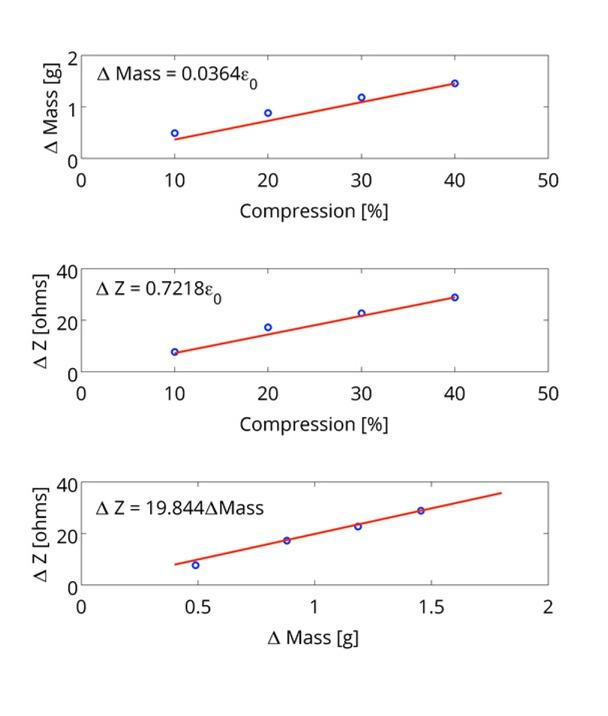The relationship between the change in mass (ΔMass), the change in impedance (ΔZ), and the compression (ε0) were found to be linear (R2 > 0.95) across all samples indicating that the change in impedance is linked with the fluid exiting the sample, which in turn is a function of compression. The values reported here were found by taking the initial and final masses and impedances of the samples.
Mechanical properties

A moving average fitting technique was used to smooth the measured stress data. From this filtered data, the stress of each run was averaged within compression levels (each with twelve trials) and plotted against time (Fig. 5). To show that no irregularities were observed for different levels of compression, the data presented in Fig. 5 was normalized to the maximum stress. These normalized values were then averaged together and a relaxation curve was fit to the Maxwell model of viscoelasticity using MATLAB (as seen in Fig. 6). Within the range of strain levels chosen, the relaxation mechanism of the tofu appears to be consistent for all samples. The corresponding equation describing the normalized stress relaxation curve – characterizing how tofu relieves stress under a constant strain – can be found in Fig. 6. While no significant variation was found for the stress relaxation time constant, the normalized elastic modulus is modified by the peak stress induced (as seen in Eq. (8)). As such, the relationship between peak stress and initial strain was found and is reported in Fig. 7. A simple linear relationship was assumed as nonlinearity of this sort is beyond the scope of the simple model we chose.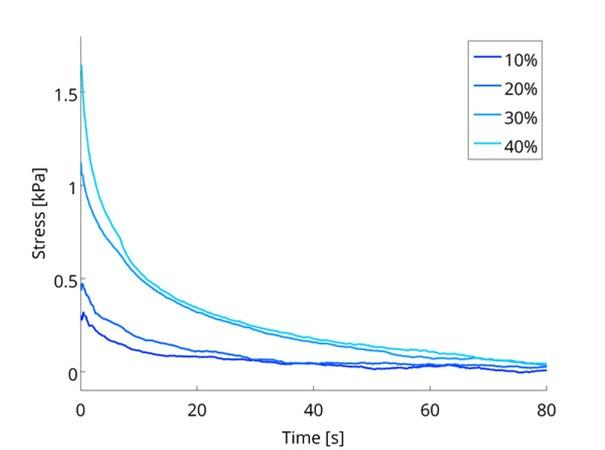Stress vs. time at four levels of compression. Using a standard moving average fitting technique the stress measurements were smoothed out and averaged within compression levels (n = 12). The initial time is set to the moment when the linear stage ceases to compress the samples.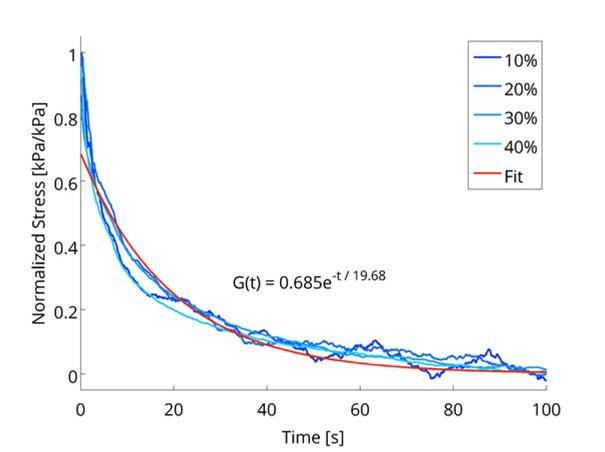The relaxation curve of the samples as represented by normalized stress vs. time plot. The stress has been normalized through the full range of values by finding the ratio of the values to the peak value observed at the initial point of relaxation. The graph highlights the relaxation mechanism of tofu observed is essentially the same in all samples.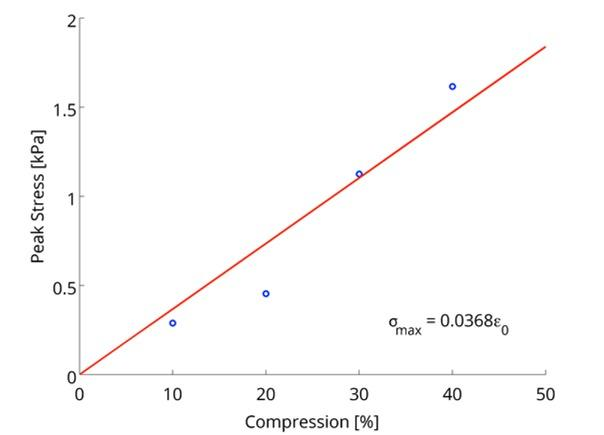Peak stress (σmax) as a function of initial compression. The relationship was found to be approximately linear and was fit via linear regression (R2 = 0.91). Given the assumptions of the chosen model, this result is in line with what was expected.

Combining the function relating peak stress to initial strain (Fig. 7) to the normalized stress relaxation curve (Eq. (8), Fig. 6) an overall viscoelastic model of tofu as a function of initial strain was found to be

$σ(t,ε0)=0.0252ε0e−t/19.68$$$\sigma \left( t,{{\varepsilon }_{0}} \right)=0.0252{{\varepsilon }_{0}}{{e}^{{-t}/{19.68}\;}}$$

where Eσ = 0.0252ε0 kPa nd τR = 19.68 s. The relative uncertainties of each of the relationships used to arrive at Eq. (9) should be kept in the reader’s mind since they potentially account for additional uncertainties as Eq. (9) is used in other contexts. The strain dependence of the stress relaxation curve begins to falter at higher initial strains, as tofu begins to shear above a certain level of compression, and thus is only described by the Maxwell viscoelasticity model for compressions <50%. These complications will be addressed in the Discussion section of the paper.

Electrical properties

As shown in Fig. 8(a), there is a trend of increasing impedance with respect to time for samples under compression. Conversely, the admittance tends to decrease over time as the sample relaxes (Fig. 8(b)). An increased level of compression results in a corresponding increase in resting impedance (the impedance when stress goes to zero), while producing a decrease in resting admittance (the admittance when stress goes to zero).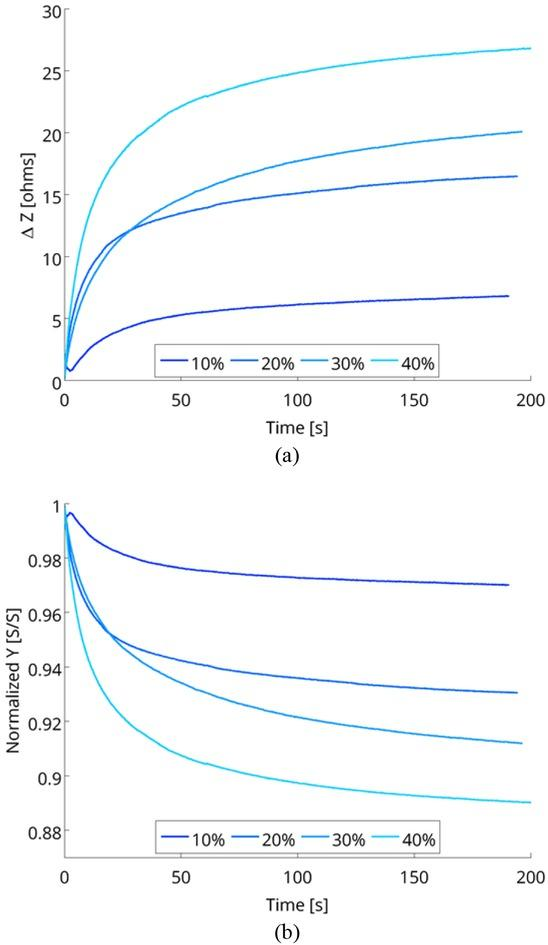The (a) average change in impedance over time and the (b) admittance normalized to the maximum value over time seen for each level of compression. Twelve individual runs comprise each of the curves. Time was set to zero at the point compression ceased.

As an initial indication of the correlation between the passive electrical properties of tofu and its viscoelastic properties, admittance was plotted as a function of stress in Fig. 9. Each admittance curve (representing the average admittance of the twelve samples tested at a particular compression level) was fit through linear regression. Two different tendencies were experienced by two parameters (intercept and slope) with respect to the strain level. The change seen in the intercept parameter verifies a result of Fig. 8 that indicated the resting admittance decreased with increasing initial strain (normalized admittance after approximately 200 s of initial compression is essentially equivalent to resting admittance). Increasing compression levels also led to a decrease in the slope parameter, indicating the strain dependence of the elasticity.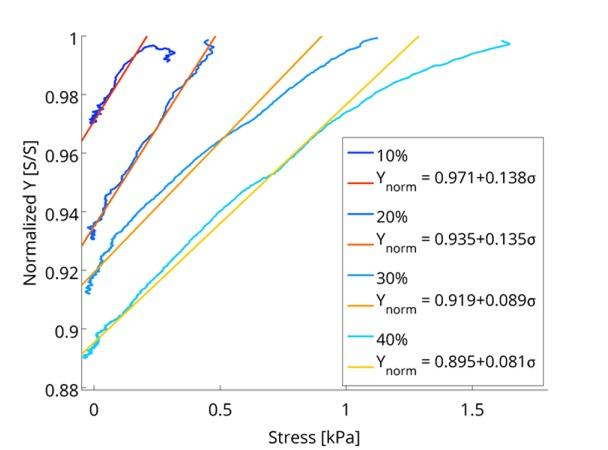Normalized admittance plotted as a function of stress and linear regression fit for each strain level. Also presented are the equations of the fit data.

The admittance vs. stress vs. time plot of Fig. 10 shows more explicitly the relationship between the stress and admittance over time for each strain level. From this alone a relationship between admittance and stress at a given time and for a given strain can be established. As further evidence of this relationship, admittance was then fit to a similar relaxation function as the stress. However, unlike stress, the normalized admittance of the tofu never falls to zero, instead settling on some resting admittance (denoted as Ynorm,Resting), requiring that we define the admittance relaxation function, a function that combines both electrical and mechanical parameters, as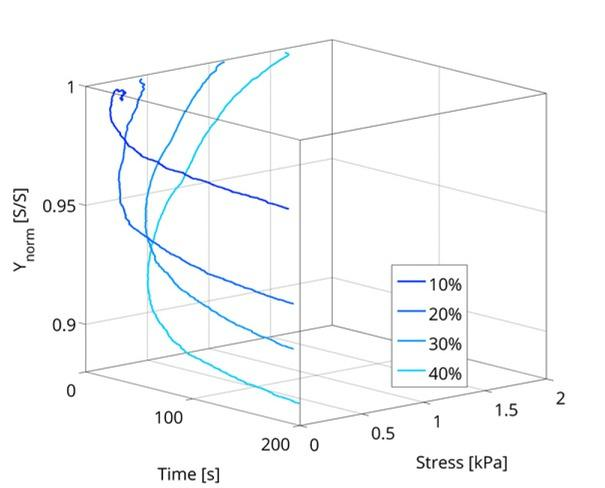Admittance vs. stress vs. time for four strain levels. The correlation between admittance and stress comes more sharply into focus when viewed in this manner.$Yt,ε0=Ynorm,Resting+EYε0e−t/τR,Y$$$\text{Y}\left( t,{{\varepsilon }_{0}} \right)={{\text{Y}}_{\text{norm},\,\text{Resting}}}+{{E}_{Y}}{{\varepsilon }_{0}}{{e}^{{-t}/{{\tau_{R}},\,Y}\;}}$$

How resting admittance changes as a function of strain is necessary to accurately fitting a relaxation curve. The planar representation seen in Fig. 9 of the multidimensional relationship between admittance, stress, strain, and time (from Fig. 10) yields the resting admittance values to formally fit the admittance curve to the hypothesize stress relaxation function. Plotting the resting admittance as a function of time (as in Fig. 11) a strain dependent relationship was established: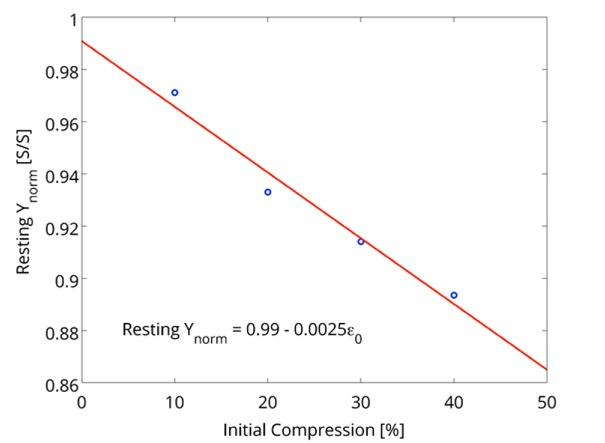Resting admittance (normalized) as a function of strain. This relationship was necessary for the admittance relaxation curve proposed in Eq. (12).$Ynorm, Resting=0.99−0.0025ε0.$$${{Y}_{\text{norm},\,\text{Resting}}}=0.99-0.0025{{\varepsilon }_{0}}.$$

The resting admittance of a sample under no strain should be equal to 1.0 in a normalized system. The 0.99 reported here is a result of empirical fitting, and was deemed to be close enough to warrant continued use. Substituting Eq. (11) into Eq. (10) yields

$Yt,ε0=0.99−0.0025−EYe−t/τR,Yε0$$$Y\left( t,{{\varepsilon }_{0}} \right)=0.99-\left( 0.0025-{{E}_{Y}}{{e}^{{-t}/{{\tau_{R}},Y}\;}} \right){{\varepsilon }_{0}}$$

Utilizing the adjusted relaxation function of Eq. (12) the admittance with respect to time were fit to an adjusted relaxation function. The goodness of fit for each of the trials was measured through the coefficient of determination (R2) and found to be greater than 0.95 in every case, suggesting the modified admittance equation correlates well to the data. This fit of the data and the average behavior of the admittance and stress for each level of strain as a response to time can be seen in Fig. 12, which suggests a relationship between admittance, stress, and strain.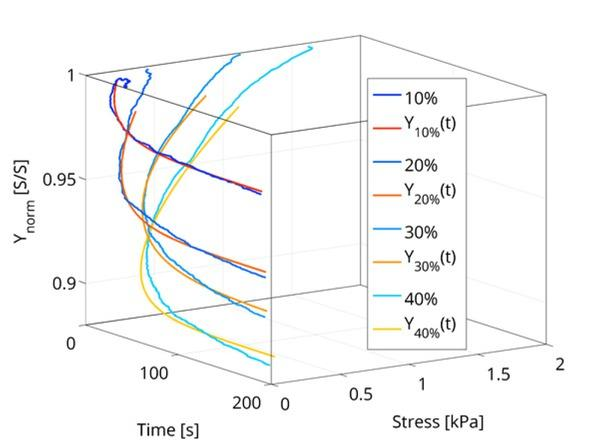Admittance vs. stress vs. time for each strain level and portions of their corresponding relaxation curves. Though the fit is not perfect, it is an appropriate first approximation that yields a high level of correlation (R2 > 0.95 in all cases).

The relaxation parameters (EY = 0.0023 ± 0.00019 and τR,Y = 29.45 ± 3.01) for admittance (like their corresponding stress counterparts) were found to be constant throughout each of the levels of strain tested. However, these variables are slightly misleading, as we have introduced a pair of concepts (an elastic modulus for admittance, EY, and a time constant of admittance relaxation, τR,Y) for which there is no basis aside from mathematical convenience. However, EY and τR,Y can be expressed wholly in terms of mechanical deformation by postulating:

$EYEσ=f(ε0)$$$\frac{{{E}_{Y}}}{{{E}_{\sigma }}}=f\left( {{\varepsilon }_{0}} \right)$$$τR,YτR,σ=gε0$$$\frac{{\tau_{R,Y}}}{{\tau_{R,\sigma }}}=g\left( {{\varepsilon }_{0}} \right)$$

where f(ε0) and g(ε0) are functions of the initial strain. This would modify Eq. (12) to become

$Yt,ε0=0.99−0.0025−fε0Eσe−t/gε0τR,σε0.$\begin{align}& Y\left( t,{{\varepsilon }_{0}} \right)=0.99-\left( 0.0025 \right. \\ & \left. -f\left( {{\varepsilon }_{0}} \right){{E}_{\sigma }}{{e}^{{-t}/{g\left( {{\varepsilon }_{0}} \right){\tau_{R,\sigma }}}\;}} \right){{\varepsilon }_{0}}. \\ \end{align}

Because the functions in these particular cases were constants (f(ε0) = 0.0091 and g(ε0) = 1.50), conversion of the dummy parameters into their mechanical equivalents was found and could be substituted into Eq. (15) to give an overall model of admittance based on the mechanical properties of tofu:

$Yt,ε0=0.99−0.0025−0.0091Eσe−t/1.50τR,σε0.$\begin{align}& Y\left( t,{{\varepsilon }_{0}} \right)=0.99-\left( 0.0025 \right. \\ & \left. -0.0091{{E}_{\sigma }}{{e}^{{-t}/{1.50{\tau_{R,\sigma }}}\;}} \right){{\varepsilon }_{0}}. \\ \end{align}

From the mechanical model proposed in Eq. (9) and the admittance model proposed in Eq. (16), we can plot the relationships of normalized admittance as a function of time and initial strain (Fig. 13(a)), normalized admittance as a function of time and stress (Fig. 13(b)), stress as a function of time and initial strain (Fig. 13(c)), and normalized admittance as a function of initial strain and stress (Fig. 13(d)). These relationships, in addition to the others used to derive them, sufficiently describe the mechanical and passive electrical behavior of tofu.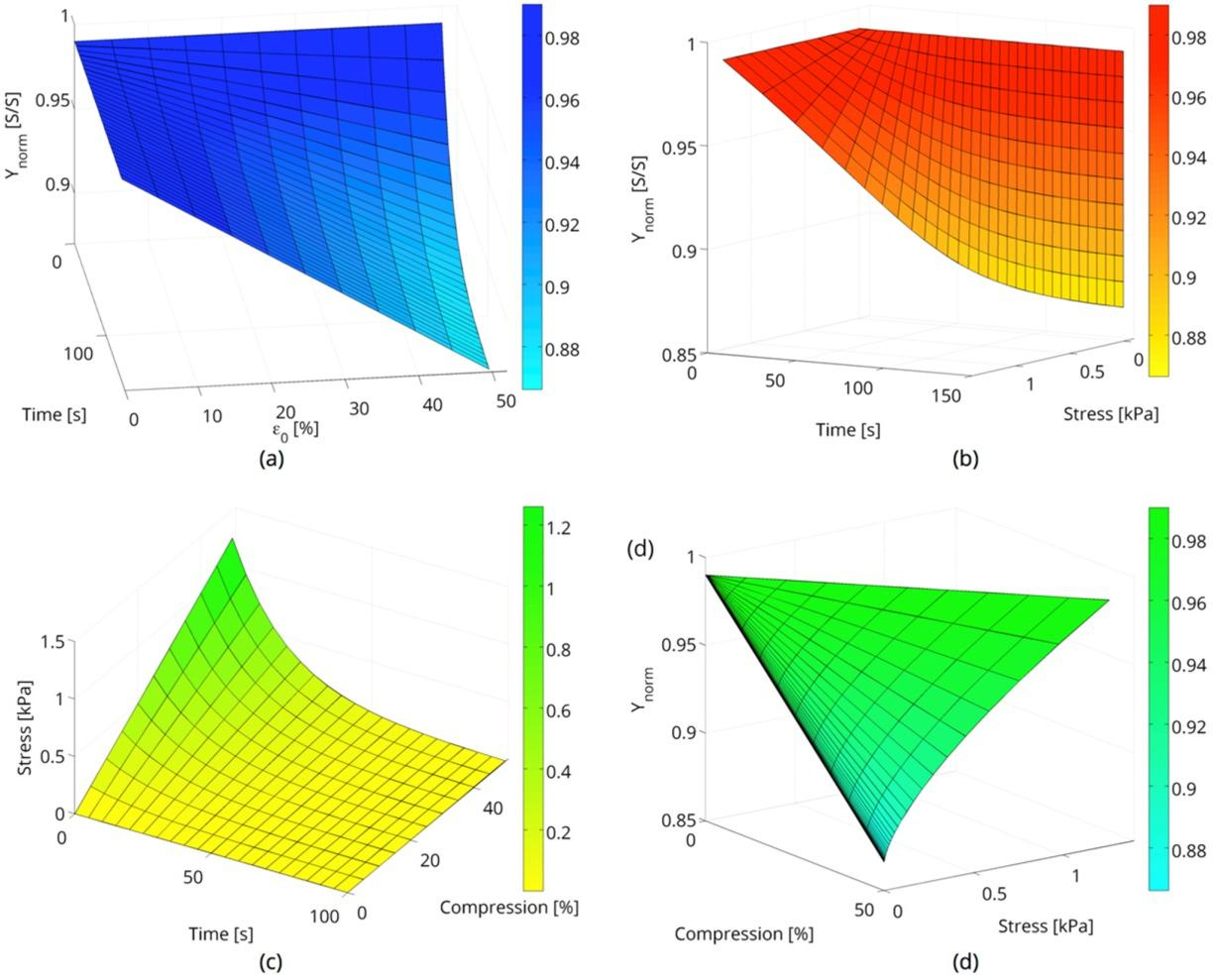The empirical model derived for (a) normalized admittance as a function of time and initial strain, (b) normalized admittance as a function of time and stress, (c) stress as a function of time and compression, and (d) normalized admittance as function of stress. Each relationship shown here fit the data well (in all cases R2 > 0.95). From empirical models presented above and the theoretical modeling presented in equations (), the underlying physical changes of the phantom can be determined and predicted with a high degree of certainty through passive electric properties measured via impedance techniques.
Discussion

The results indicate that the mechanical changes experienced by the soft tissue-mimicking phantom material (tofu) correlate well to the measured impedance as a sample is compressed. The link between mechanical strain and electrical admittance is established in this research. We have shown: (1) that impedance is determined by the amount of conductive fluid within a porous material and to what degree the material is compressed (Fig. 4); (2) the viscoelastic behavior of tofu described well (R2 > 0.95) through a range of compression levels (Fig. 5 and 6) resulting in a mechanical model of tofu (Eq. (9)); (3) the behavior of normalized admittance correlating well with initial strain level, resulting from a simple constant relationship with the stress relaxation curve (Fig. 9Fig. 12); and (4) a final physical model of tofu encapsulating both mechanical and passive electrical properties (Fig. 13 and Eq. 18). This culmination of facts shows that tofu is capable of testing the predictions made by researchers about the effects of pressure and force on measured bioimpedance (19,21-23).

While the above stated evidence is on a firm foundation and provide sufficient support to justify further examination of tofu as a phantom material able to mimic the relevant behavior of tissue under compression, there are many ways in which this study here can be further improved. Two general modes exist for this improvement and can be thought of as modeling improvements of mechanical properties and further empirical examination of the experimental parameters.

Modeling improvements

Improvements to modeling can be broken down into two significant subsets. The first place where model improvement would help nuance the claims made here is through proper modeling of tofu as a soft biological material. For a mixture of simplicity and expediency the Maxwell model of viscoelasticity was used to predict the stress relaxation of tofu, though this need not be the case. Another standard model that might possibly enhance the predictions is the Kelvin-Voigt model of viscoelasticity. It is similar to the Maxwell model in that its mechanical analog consists simply of a spring and damper (dashpot), but rather than attaching the two in series, they are in parallel with one another. This provides the subtle difference in how displacement of the two elements are added together (for the Maxwell model they are simply summed, for Kelvin-Voigt they are equal). Therefore, because the strain of the spring and damper analogues are equal, the stress through each element is now separate, making the total stress of the system equivalent to their sum. Where the Kelvin-Voigt model excels over the Maxwell model is its more realistic prediction of creep, a neglected factor in this study of possible significance.

Another model that has been considered for later publication is the quasi-linear viscoelastic theory first proposed by Fung (7) and further expounded at length by Abramowitch and Woo (41) which is normally used for biological materials with marked history dependence. It is a significant improvement over the previous two models as it introduces history dependence and splits the test into two distinct regions (during the initial ramp up of compression and after this ramp up). In so doing, this model accounts for the reality of the situation to a greater degree than either of the two models discussed and will likely fit the stress relaxation data more precisely.

Further refinement could be addressed in the modeling of the impedance of tofu as well. What was developed here was a simple relationship between the relative contributions of each components resistance to the total. Such a model proved useful here as it predicted that with the exodus of the less resistant component, the overall impedance of the sample would increase. Said another way, as the more conductive fluid was forced out given its incompressibility, the admittance of the sample should decrease – it should decrease more and more as the applied compression is increased. One drawback of this modeling approach is the difficulty of measuring it accurately with the setup presented here (consult the next section for how this may be improved). An avenue of rich potential is a model that treats impedance as a more explicit function of fluid being pushed through a compressing porous structure.

Further Empirical Examination

One key parameter of this study was measured rather indirectly and could be improved upon, namely the loss of fluid with respect to time. The fluid loss in this experiment was measured by taking mass readings before and after the compressive runs. Such a method only gives two points of examination for changes in relative density. While the evidence presented is promising to validate the proposed model of compressed tofu impedance, it is in need of more points of verification. A simple way of providing this verification is with the addition of a flowmeter to the experimental setup capable of tracking the amount of fluid that has accumulated at any particular point in time.

Finally, these tests should be replicated in tissue, where complex impedance is seen. A multifrequency technique would be necessary to measure the complex impedance in real-time, a problem of many frequency sweep procedures currently. Only such a system would be able to trace both the intra- and extra-cellular changes that occur for tissues under compression.

Conclusions

A non-invasive electrical impedance measurement system was developed, calibrated, and verified to accurately measure the passive electrical properties of tofu under physical deformation. This was accomplished by examining the stresses, strains, electrical impedance, and changes in density of tofu – a quasi-biological tissue-mimicking material – while it was subjected to compression. We have endeavored to show that tofu is a suitable phantom material for consideration for experiments seeking to correlate impedance measurements to tissue compression. Though the experiments described within were simple – tracking the impedance of tofu as it was compressed – the underlying phenomena were complex.

The physical properties associated with the deformation (be it made visible through viscoelastic theory or measured passive electrical components) have relevance to many physiological, pathological, clinical, and basic research-based systems. As such it is our hope that this initial exploration of a broader field investigating the correlative effects of tissue deformation and electrical impedance in need of rigorous and multidisciplinary consideration will spark the interest of a few.

Sun T, Gawad S, Bernabini C, Green NG, Morgan H. Broadband single cell impedance spectroscopy using maximum length sequences: theoretical analysis and practical considerations. Meas. Sci. Technol. 2007 Jul.20;18(9):2859–68. http://dx.doi.org/10.1088/0957-0233/18/9/01510.1088/0957-0233/18/9/015SunTGawadSBernabiniCGreenNGMorganHBroadband single cell impedance spectroscopy using maximum length sequences: theoretical analysis and practical considerationsMeas. Sci. Technol2007Jul.20189285968http://dx.doi.org/10.1088/0957-0233/18/9/015

K'Owino IO, Sadik OA. Impedance Spectroscopy: A Powerful Tool for Rapid Biomolecular Screening and Cell Culture Monitoring. Electroanalysis. 2005 Dec.;17(23):2101–13. http://dx.doi.org/10.1002/elan.20050337110.1002/elan.200503371K'OwinoIOSadikOAImpedance Spectroscopy: A Powerful Tool for Rapid Biomolecular Screening and Cell Culture MonitoringElectroanalysis20051723210113http://dx.doi.org/10.1002/elan.200503371

Miklavčič D, Pavšelj N, Hart FX. Electric properties of tissues. Wiley Encyclopedia of Biomedical Engineering. Wiley Online Library; 2006.MiklavčičDPavšeljNHartFXElectric properties of tissues. Wiley Encyclopedia of Biomedical EngineeringWiley Online Library200610.1002/9780471740360.ebs0403Search in Google Scholar

Paulson KS, Pidcock MK, McLeod CN. A Probe for Organ Impedance Measurement. IEEE Trans. Biomed. Eng. 2004 Oct.;51(10):1838–44. http://dx.doi.org/10.1109/TBME.2004.83151810.1109/TBME.2004.83151815490831PaulsonKSPidcockMKMcLeodCNA Probe for Organ Impedance MeasurementIEEE Trans. Biomed. Eng20045110183844http://dx.doi.org/10.1109/TBME.2004.83151815490831

Foster KR, Lukaski HC. Whole-body impedance--what does it measure? The American journal of clinical nutrition. Am Soc Nutrition; 1996;64(3):388S–396S.FosterKRLukaskiHCWhole-body impedance--what does it measure? The American journal of clinical nutritionAm Soc Nutrition;1996643388S39610.1093/ajcn/64.3.388S8780354Search in Google Scholar

Tanabe RF, de Azevedo ZMA, Fonseca VM, Peixoto MVM, Anjos dos LA, Gaspar-Elsas MIC, et al. Distribution of bioelectrical impedance vector values in multi-ethnic infants and pre-school children. Clinical Nutrition. Elsevier Ltd; 2012 Feb.1;31(1):144–8. http://dx.doi.org/10.1016/j.clnu.2011.08.006TanabeRFde AzevedoZMAFonsecaVMPeixotoMVMAnjosdos LAGaspar-ElsasMICet alDistribution of bioelectrical impedance vector values in multi-ethnic infants and pre-school childrenClinical Nutrition. Elsevier Ltd2012Feb.13111448http://dx.doi.org/10.1016/j.clnu.2011.08.00610.1016/j.clnu.2011.08.00621872371Search in Google Scholar

Fung YC. Biomechanics: Mechanical Properties of Living Tissues. Springer-Verlag; 1993.FungYCBiomechanics: Mechanical Properties of Living TissuesSpringer-Verlag;1993Search in Google Scholar

Demou ZN. Gene Expression Profiles in 3D Tumor Analogs Indicate Compressive Strain Differentially Enhances Metastatic Potential. Ann Biomed Eng. 2010 Jun. 18;38(11):3509–20. http://dx.doi.org/10.1007/s10439-010-0097-010.1007/s10439-010-0097-020559731DemouZNGene Expression Profiles in 3D Tumor Analogs Indicate Compressive Strain Differentially Enhances Metastatic PotentialAnn Biomed Eng2010Jun. 183811350920http://dx.doi.org/10.1007/s10439-010-0097-020559731

Ingber DE. Cellular mechanotransduction: putting all the pieces together again. The FASEB journal. FASEB; 2006;20(7):811–27. http://dx.doi.org/10.1096/fj.05-5424rev10.1096/fj.05-5424revIngberDECellular mechanotransduction: putting all the pieces together againThe FASEB journal. FASEB;200620781127http://dx.doi.org/10.1096/fj.05-5424rev16675838

Knezevich BA. Trauma nursing: principles and practice. Appleton-Century-Crofts; 1986.KnezevichBATrauma nursing: principles and practiceAppleton-Century-Crofts;1986Search in Google Scholar

Berry GP, Bamber JC, Mortimer PS, Bush NL, Miller NR, Barbone PE. The Spatio-Temporal Strain Response of Oedematous and Nonoedematous Tissue to Sustained Compression In Vivo. Ultrasound in Medicine & Biology. Elsevier; 2008;34(4):617–29.10.1016/j.ultrasmedbio.2007.10.007BerryGPBamberJCMortimerPSBushNLMillerNRBarbonePEThe Spatio-Temporal Strain Response of Oedematous and Nonoedematous Tissue to Sustained Compression In VivoUltrasound in Medicine & Biology. Elsevier;20083446172918222033

Dodde RE, Miller SF, Geiger JD, Shih AJ. Thermal-Electric Finite Element Analysis and Experimental Validation of Bipolar Electrosurgical Cautery. J. Manuf. Sci. Eng. 2008;130(2):021015. http://dx.doi.org/10.1115/1.290285810.1115/1.2902858DoddeREMillerSFGeigerJDShihAJThermal-Electric Finite Element Analysis and Experimental Validation of Bipolar Electrosurgical CauteryJ. Manuf. Sci. Eng20081302021015http://dx.doi.org/10.1115/1.2902858

Khanna A, Gougoulias N, Maffulli N. Intermittent pneumatic compression in fracture and soft-tissue injuries healing. British Medical Bulletin. 2008Dec.5;88(1):147–56. http://dx.doi.org/10.1093/bmb/ldn02410.1093/bmb/ldn02418596049KhannaAGougouliasNMaffulliNIntermittent pneumatic compression in fracture and soft-tissue injuries healingBritish Medical Bulletin2008Dec.588114756http://dx.doi.org/10.1093/bmb/ldn024

Rylander CG, Stumpp OF, Milner TE, Kemp NJ, Mendenhall JM, Diller KR, et al. Dehydration mechanism of optical clearing in tissue. J. Biomed. Opt. 2006;11(4):041117. http://dx.doi.org/10.1117/1.234320810.1117/1.234320816965145RylanderCGStumppOFMilnerTEKempNJMendenhallJMDillerKRet alDehydration mechanism of optical clearing in tissueJ. Biomed. Opt2006114041117http://dx.doi.org/10.1117/1.2343208

Keshtkar A. Design and construction of small sized pencil probe to measure bio-impedance. Medical Engineering & Physics. 2007 Nov.;29(9):1043–8. http://dx.doi.org/10.1016/j.medengphy.2006.10.0101711869110.1016/j.medengphy.2006.10.010KeshtkarADesign and construction of small sized pencil probe to measure bio-impedanceMedical Engineering & Physics200729910438http://dx.doi.org/10.1016/j.medengphy.2006.10.010Search in Google Scholar

Lepetit J, Culioli J. Mechanical properties of meat. Meat Science. Elsevier; 1994;36(1-2):203–37. http://dx.doi.org/10.1016/0309-1740(94)90042-610.1016/0309-1740(94)90042-6LepetitJCulioliJMechanical properties of meatMeat Science. Elsevier;1994361-220337http://dx.doi.org/10.1016/0309-1740(94)90042-6

Lepetit J, Sale P, Favier R, Dalle R. Electrical impedance and tenderisation in bovine meat. Meat Science. Elsevier; 2002;60(151–62. http://dx.doi.org/10.1016/S0309-1740(01)00104-810.1016/S0309-1740(01)00104-8LepetitJSalePFavierRDalleRElectrical impedance and tenderisation in bovine meatMeat Science. Elsevier;20026015162http://dx.doi.org/10.1016/S0309-1740(01)00104-8

Kahraman S, Alber M. Predicting the physico-mechanical properties of rocks from electrical impedance spectroscopy measurements. International Journal of Rock Mechanics and Mining Sciences. 2006 Jun.;43(4):543–53. http://dx.doi.org/10.1016/j.ijrmms.2005.09.01310.1016/j.ijrmms.2005.09.013KahramanSAlberMPredicting the physico-mechanical properties of rocks from electrical impedance spectroscopy measurementsInternational Journal of Rock Mechanics and Mining Sciences200643454353http://dx.doi.org/10.1016/j.ijrmms.2005.09.013

González-Correa CA, Brown BH, Smallwood RH, Walker DC, Bardhan KD. Electrical bioimpedance readings increase with higher pressure applied to the measuring probe. Physiol. Meas. 2005 Mar.30;26(2):S39–S47. http://dx.doi.org/10.1088/0967-3334/26/2/00410.1088/0967-3334/26/2/00415798245González-CorreaCABrownBHSmallwoodRHWalkerDCBardhanKDElectrical bioimpedance readings increase with higher pressure applied to the measuring probePhysiol. Meas2005Mar.30262S39S47http://dx.doi.org/10.1088/0967-3334/26/2/00415798245

Tsai JZ, Cao H, Tungjitkusolmun S, Woo EJ, Vorperian VR, Webster JG. Dependence of apparent resistance of four-electrode probes on insertion depth. IEEE Trans. Biomed. Eng. IEEE; 2000;47(1):41–8. http://dx.doi.org/10.1109/10.81761810.1109/10.817618TsaiJZCaoHTungjitkusolmunSWooEJVorperianVRWebsterJGDependence of apparent resistance of four-electrode probes on insertion depthIEEE Trans. Biomed. Eng. IEEE;2000471418http://dx.doi.org/10.1109/10.81761810646278

Keshtkar A, Keshtkar A. The effect of applied pressure on the electrical impedance of the bladder tissue using small and large probes. Journal of Medical Engineering & Technology. Informa UK Ltd UK; 2008;32(6):505–11.KeshtkarAKeshtkarAThe effect of applied pressure on the electrical impedance of the bladder tissue using small and large probesJournal of Medical Engineering & Technology. Informa UK Ltd UK;20083265051110.1080/0309190070150745619005965Search in Google Scholar

Keshtkar A, Keshtkar A. Probe pressure optimisation in bio-impedance spectroscopy. International Journal of Medical Engineering and Informatics. Inderscience; 2011;3(1):78–83.10.1504/IJMEI.2011.039078KeshtkarAKeshtkarAProbe pressure optimisation in bio-impedance spectroscopyInternational Journal of Medical Engineering and Informatics. Inderscience;2011317883

Dodde RE, Bull JL, Shih AJ. Bioimpedance of soft tissue under compression. Physiol. Meas. 2012 May 24;33(6):1095–109. http://dx.doi.org/10.1088/0967-3334/33/6/109510.1088/0967-3334/33/6/109522621935DoddeREBullJLShihAJBioimpedance of soft tissue under compressionPhysiol. Meas2012May 243361095109http://dx.doi.org/10.1088/0967-3334/33/6/109522621935

Righetti R, Ophir J, Srinivasan S, Krouskop TA. The feasibility of using elastography for imaging the Poisson's ratio in porous media. Ultrasound in Medicine & Biology. 2004 Feb.;30(2):215–28. http://dx.doi.org/10.1016/j.ultrasmedbio.2003.10.0221499867410.1016/j.ultrasmedbio.2003.10.022RighettiROphirJSrinivasanSKrouskopTAThe feasibility of using elastography for imaging the Poisson's ratio in porous mediaUltrasound in Medicine & Biology200430221528http://dx.doi.org/10.1016/j.ultrasmedbio.2003.10.02214998674Search in Google Scholar

Kim YT, Kim HC, Inada-Kim M, Jung SS, Yun YH, Jho MJ, et al. Evaluation of Tissue Mimicking Quality of Tofu for Biomedical Ultrasound. UMB. World Federation for Ultrasound in Medicine & Biology; 2009 Mar.1;35(3):472–81. http://dx.doi.org/10.1016/j.ultrasmedbio.2008.09.00510.1016/j.ultrasmedbio.2008.09.005KimYTKimHCInada-KimMJungSSYunYHJhoMJet alEvaluation of Tissue Mimicking Quality of Tofu for Biomedical UltrasoundUMB. World Federation for Ultrasound in Medicine & Biology;2009Mar.135347281http://dx.doi.org/10.1016/j.ultrasmedbio.2008.09.00519101073

Aguilera JM, Stanley DW. Microstructural principles of food processing and engineering. Springer; 1999.AguileraJMStanleyDWMicrostructural principles of food processing and engineeringSpringer;1999Search in Google Scholar

Li X, Toyoda K, Ihara I. Coagulation process of soymilk characterized by electrical impedance spectroscopy. Journal of Food Engineering. Elsevier Ltd; 2011 Aug. 1;105(3):563–8. http://dx.doi.org/10.1016/j.jfoodeng.2011.03.023LiXToyodaKIharaICoagulation process of soymilk characterized by electrical impedance spectroscopyJournal of Food Engineering. Elsevier Ltd;2011Aug. 110535638http://dx.doi.org/10.1016/j.jfoodeng.2011.03.02310.1016/j.jfoodeng.2011.03.023Search in Google Scholar

Li XS, Toyoda K. Monitoring of the coagulation process of soymilk by an integrated electrical sensing and control system. Mathematical and Computer Modelling. Elsevier Ltd; 2011 Dec.2;:1–8.LiXSToyodaKMonitoring of the coagulation process of soymilk by an integrated electrical sensing and control systemMathematical and Computer Modelling. Elsevier Ltd;2011Dec. 21810.1016/j.mcm.2011.11.001Search in Google Scholar

Wu J. Tofu as a tissue-mimicking material. UMB. Elsevier; 2001;27(9):1297–300.WuJTofu as a tissue-mimicking materialUMB. Elsevier20012791297300Search in Google Scholar

Christensen RM. Theory of viscoelasticity. Dover Publications; 2010.ChristensenRMTheory of viscoelasticityDover Publications;201010.1115/1.3408900Search in Google Scholar

Stogryn A. Equations for calculating the dielectric constant of saline water (Correspondence). Microwave Theory and Techniques, IEEE Transactions on. IEEE; 1971;19(8):733–6.10.1109/TMTT.1971.1127617StogrynAEquations for calculating the dielectric constant of saline water (Correspondence)Microwave Theory and Techniques, IEEE Transactions on. IEEE;19711987336

Li X, Toyoda K. Monitoring of coagulation process of soymilk by an integrated electrical sensing and control system. Mathematical and Computer Modelling. Elsevier; 2011.LiXToyodaKMonitoring of coagulation process of soymilk by an integrated electrical sensing and control systemMathematical and Computer Modelling. Elsevier;201110.1016/j.mcm.2011.11.001Search in Google Scholar

Dodde R. Bioimpedance of soft tissue under compression and applications to electrosurgery. PhD Thesis. University of Michigan, USA, 2012.DoddeRBioimpedance of soft tissue under compression and applications to electrosurgeryPhD ThesisUniversity of MichiganUSA201210.1088/0967-3334/33/6/1095Search in Google Scholar

Brown B, Wilson A, Bertemes-Filho P. Bipolar and tetrapolar transfer impedance measurements from volume conductor. Electronics Letters. IET; 2000;36(25):2060–2.10.1049/el:20001439BrownBWilsonABertemes-FilhoPBipolar and tetrapolar transfer impedance measurements from volume conductorElectronics Letters. IET2000362520602

Grimnes S, Martinsen ØG. Sources of error in tetrapolar impedance measurements on biomaterials and other ionic conductors. J. Phys. D: Appl. Phys. 2006 Dec.15;40(1):9–14. http://dx.doi.org/10.1088/0022-3727/40/1/S02GrimnesSMartinsenØGSources of error in tetrapolar impedance measurements on biomaterials and other ionic conductorsJ. Phys. D: Appl. Phys2006Dec.15401914http://dx.doi.org/10.1088/0022-3727/40/1/S0210.1088/0022-3727/40/1/S02Search in Google Scholar

Scharfetter H, Hartinger P, Hinghofer-Szalkay H, Hutten H. A model of artefacts produced by stray capacitance during whole body or segmental bioimpedance spectroscopy. Physiol. Meas. IOP Publishing; 1998;19:247. http://dx.doi.org/10.1088/0967-3334/19/2/01210.1088/0967-3334/19/2/012ScharfetterHHartingerPHinghofer-SzalkayHHuttenHA model of artefacts produced by stray capacitance during whole body or segmental bioimpedance spectroscopyPhysiol. Meas. IOP Publishing;199819247http://dx.doi.org/10.1088/0967-3334/19/2/0129626689

Bolton M, Ward L, Khan A, Campbell I, Nightingale P, Dewit O, et al. Sources of error in bioimpedance spectroscopy. Physiol. Meas. IOP Publishing; 1998;19:235. http://dx.doi.org/10.1088/0967-3334/19/2/01110.1088/0967-3334/19/2/011BoltonMWardLKhanACampbellINightingalePDewitOet alSources of error in bioimpedance spectroscopyPhysiol. Meas. IOP Publishing199819235http://dx.doi.org/10.1088/0967-3334/19/2/0119626688

McEwan A, Cusick G, Holder DS. A review of errors in multi-frequency EIT instrumentation. Physiol. Meas. 2007 Jun.26;28(7):S197–S215. http://dx.doi.org/10.1088/0967-3334/28/7/S151766463610.1088/0967-3334/28/7/S15McEwanACusickGHolderDSA review of errors in multi-frequency EIT instrumentationPhysiol. Meas2007Jun.26287S197S215http://dx.doi.org/10.1088/0967-3334/28/7/S1517664636Search in Google Scholar

Buendia R, Seoane F, Gil-Pita R. A novel approach for removing the hook effect artefact from Electrical Bioimpedance spectroscopy measurements. J. Phys.: Conf. Ser. 2010 May19;224:012126.BuendiaRSeoaneFGil-PitaRA novel approach for removing the hook effect artefact from Electrical Bioimpedance spectroscopy measurementsJ. Phys.: Conf. Ser201022401212610.1088/1742-6596/224/1/012126Search in Google Scholar

Buendia R, Seoane F, Gil-Pita R. Experimental validation of a method for removing the capacitive leakage artifact from electrical bioimpedance spectroscopy measurements. Meas. Sci. Technol. 2010 Oct.6;21(11):115802. http://dx.doi.org/10.1088/0957-0233/21/11/11580210.1088/0957-0233/21/11/115802BuendiaRSeoaneFGil-PitaRExperimental validation of a method for removing the capacitive leakage artifact from electrical bioimpedance spectroscopy measurementsMeas. Sci. Technol2010Oct.62111115802http://dx.doi.org/10.1088/0957-0233/21/11/115802

Abramowitch SD, Woo SLY. An Improved Method to Analyze the Stress Relaxation of Ligaments Following a Finite Ramp Time Based on the Quasi-Linear Viscoelastic Theory. J. Biomech. Eng. 2004;126(1):92. http://dx.doi.org/10.1115/1.16455281517113410.1115/1.1645528AbramowitchSDWooSLYAn Improved Method to Analyze the Stress Relaxation of Ligaments Following a Finite Ramp Time Based on the Quasi-Linear Viscoelastic TheoryJ. Biomech. Eng2004126192http://dx.doi.org/10.1115/1.164552815171134Search in Google Scholar

Articles recommandés par Trend MD